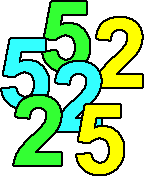#### You may also like### Multiplication Magic

Given any 3 digit number you can use the given digits and name another number which is divisible by 37 (e.g. given 628 you say 628371 is divisible by 37 because you know that 6+3 = 2+7 = 8+1 = 9). The question asks you to explain the trick.### Transposition Fix

Suppose an operator types a US Bank check code into a machine and transposes two adjacent digits will the machine pick up every error of this type? Does the same apply to ISBN numbers; will a machine detect transposition errors in these numbers?### Check Codes

Details are given of how check codes are constructed (using modulus arithmetic for passports, bank accounts, credit cards, ISBN book numbers, and so on. A list of codes is given and you have to check if they are valid identification numbers?

# Fac-finding

##### Age 14 to 16 Challenge Level:If factorial $100$ ($100!$) was rewritten as the product of its prime factors how many $2$s and how many $5$s would there be?

Along the way, confirm how many zeros are at the end of this large number and what the first digit to precede them is.

In the Teachers' Resources, Lyndon explains why he likes this problem.